DRV8302 - High performance BLDC driver using Arduino UNO

DRV8302 is a high performance BLDC driver board capable of delivering 15A current continuously and 27A peak current. It has temperature and over-current protection, it has Back EMF and three phase current sensing and last but not the least it can be run using only 3PWM signals making it compatible with the SimpleFOClibrary.

BEWARE📢

Up to this moment (version 1.4.1 ) the library doesn't implement the current control loop. The motor torque is controlled via voltage directly. More info.

Here is an example hardware you might need for this project:

Arduino UNODRV8302AMT 103 encoderGBM5108-120T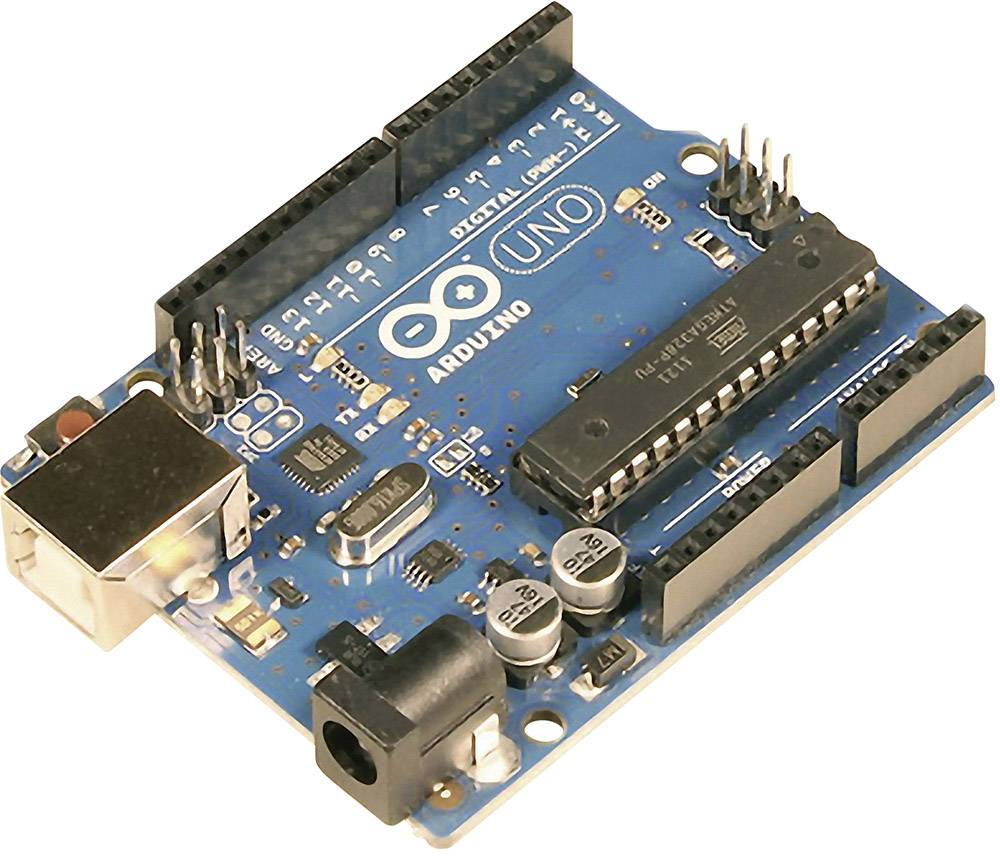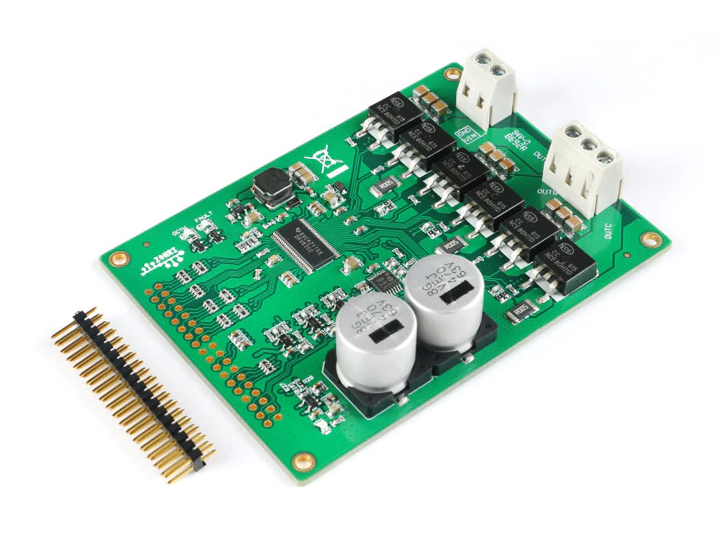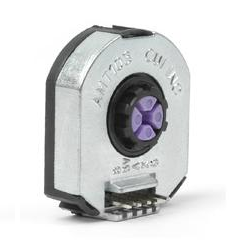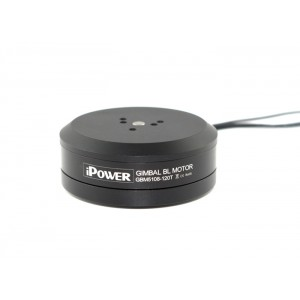Here is an example of the connection scheme using the Arduino UNO:DRV8302

• As any other BLDC driver supported with this library the board receives 3PWM signals: pwm a,b and c. Connect them to INHA, INHB and INHC
• Additionally connect the enable pin as well to EN-GATE pin
• To configure the BLDC driver we will need three pins (purple)
• M_PWM when high enables 3PWM mode (if low the board expects 6PWM signals)
• M_OC when low enables over-current protection
• To read the fault signals we will need to read two pins (red)
• nFAULT when in high board in fault state
• nOCTW when in high over-current limit reached

Encoder

• Channels A and B are connected to the external interrupt pins 2 and 3
• If your encoder has index signal you can connect it to any available digital pin, here we connected it to the pin 4

Motor

• Motor phases a, b and c are connected directly the board terminals OUTA, OUTB and OUTC

Example connection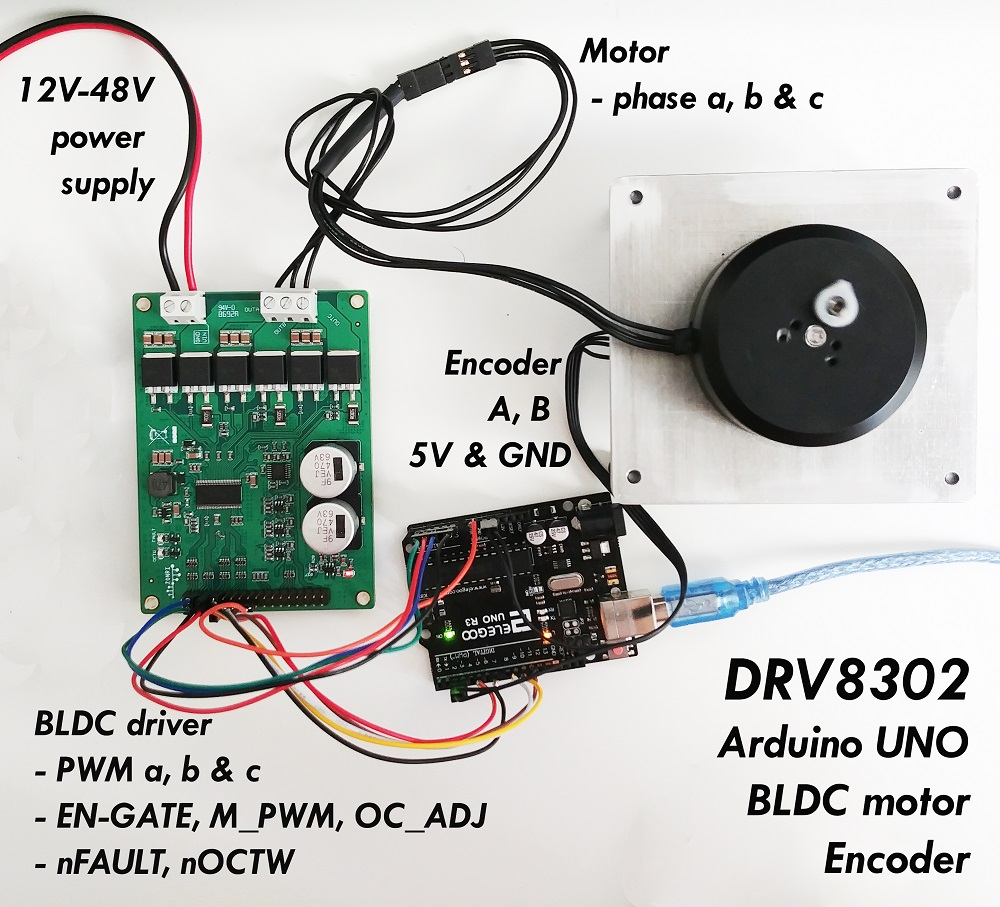Arudino code 3PWM

The code to run the DRV8302 board with 3PWM is almost exactly the same as for the other low-power BLDC drivers. The only difference is the configuration procedure of the DRV8302.

Therefore let’s first start with the definitions of pins we are using with the board:

// DRV8302 pins connections
// don't forget to connect the common ground pin
#define INH_A 9
#define INH_B 10
#define INH_C 11
#define EN_GATE 8
#define M_PWM 6
#define M_OC 5

The variables INH_A, INH_B, INH_C and EN_GATE are supplied directly to the BLDCDriver3PWM class constructor.:

// driver instance
BLDCDriver3PWM driver = BLDCDriver3PWM(INH_A, INH_B, INH_C, EN_GATE);

The only other DRV8302 specific part of the code you need to do, is to add on the beginning of the setup() function:

// DRV8302 specific code
// M_OC  - enable over-current protection
pinMode(M_OC,OUTPUT);
digitalWrite(M_OC,LOW);
// M_PWM  - enable 3pwm mode
pinMode(M_PWM,OUTPUT);
digitalWrite(M_PWM,HIGH);
// OD_ADJ - set the maximum over-current limit possible
// Better option would be to use voltage divisor to set exact value

And the rest is the regular SimpleFOClibrary code for running BLDC motors.

Here is the full code of this project:

#include <SimpleFOC.h>

// DRV8302 pins connections
// don't forget to connect the common ground pin
#define INH_A 9
#define INH_B 10
#define INH_C 11
#define EN_GATE 8
#define M_PWM 6
#define M_OC 5

// motor instance
BLDCMotor motor = BLDCMotor(11);

// driver instance
BLDCDriver3PWM driver = BLDCDriver3PWM(INH_A, INH_B, INH_C, EN_GATE);

// encoder instance
Encoder encoder = Encoder(2, 3, 8192);
// channel A and B callbacks
void doA(){encoder.handleA();}
void doB(){encoder.handleB();}

// commander interface
Commander command = Commander(Serial);
void onMotor(char* cmd){ command.motor(&motor, cmd); }

void setup() {

// initialize encoder sensor hardware
encoder.init();
encoder.enableInterrupts(doA, doB);
// link the motor to the sensor

// DRV8302 specific code
// M_OC  - enable over-current protection
pinMode(M_OC,OUTPUT);
digitalWrite(M_OC,LOW);
// M_PWM  - enable 3pwm mode
pinMode(M_PWM,OUTPUT);
digitalWrite(M_PWM,HIGH);
// OD_ADJ - set the maximum over-current limit possible
// Better option would be to use voltage divisor to set exact value

// configure driver
driver.voltage_power_supply = 12;
driver.init();

// choose FOC modulation
motor.foc_modulation = FOCModulationType::SpaceVectorPWM;

// set control loop type to be used
motor.controller = MotionControlType::torque;

// controller configuration based on the control type
motor.PID_velocity.P = 0.2;
motor.PID_velocity.I = 20;

// velocity low pass filtering time constant
motor.LPF_velocity.Tf = 0.01;

// angle loop controller
motor.P_angle.P = 20;

// angle loop velocity limit
motor.velocity_limit = 50;
// default voltage_power_supply
motor.voltage_limit = 12;

// use monitoring with serial for motor init
// monitoring port
Serial.begin(115200);
// comment out if not needed
motor.useMonitoring(Serial);

// initialize motor
motor.init();
// align encoder and start FOC
motor.initFOC();

// set the initial target value
motor.target = 2;

// define the motor id

_delay(1000);
}

void loop() {
// iterative setting FOC phase voltage
motor.loopFOC();

// iterative function setting the outer loop target
// velocity, position or voltage
// if target not set in parameter uses motor.target variable
motor.move();

// user communication
command.run();
}

Arduino code 6PWM

The code to run the DRV8302 board with 6PWM we need to be careful to configure the pins correctly based on the microcontroller we are using.

An example fo Arduino uno pinout can be:

// DRV8302 pins connections
// don't forget to connect the common ground pin
#define INH_A 5
#define INH_B 9
#define INH_C 11
#define INL_A 6
#define INL_B 10
#define INL_C 3
#define EN_GATE 8
#define M_PWM 6
#define M_OC 5

For stm32 Nucleo board that pinout would not be suitable but this one would work:

// DRV8302 pins connections
// don't forget to connect the common ground pin
#define INH_A 7
#define INH_B 6
#define INH_C 5
#define INL_A 2
#define INL_B 3
#define INL_C 4
#define EN_GATE 8
#define M_PWM 9
#define M_OC 10

The variables INH_A, INH_B, INH_C,INL_A, INL_B, INHL_C and EN_GATE are supplied directly to the BLDCDriver6PWM class constructor.:

// driver instance
BLDCDriver6PWM driver = BLDCDriver6PWM(INH_A, INL_A, INH_B,INL_B, INH_C, INL_C, EN_GATE);

The only other DRV8302 specific part of the code you need to do, is to add on the beginning of the setup() function:

// DRV8302 specific code
// M_OC  - enable over-current protection
pinMode(M_OC,OUTPUT);
digitalWrite(M_OC,LOW);
// M_PWM  - enable 6pwm mode (can be left open)
pinMode(M_PWM,OUTPUT);
digitalWrite(M_PWM,LOW);
// OD_ADJ - set the maximum over-current limit possible
// Better option would be to use voltage divisor to set exact value

And the rest is the regular SimpleFOClibrary code for running BLDC motors.

Here is the full code of this project:

#include <SimpleFOC.h>

// DRV8302 pins connections
// don't forget to connect the common ground pin
#define INH_A 5
#define INH_B 9
#define INH_C 11
#define INL_A 6
#define INL_B 10
#define INL_C 3
#define EN_GATE 8
#define M_PWM 6
#define M_OC 5

// motor instance
BLDCMotor motor = BLDCMotor(11);

// driver instance
BLDCDriver6PWM driver = BLDCDriver6PWM(INH_A, INL_A, INH_B,INL_B, INH_C, INL_C, EN_GATE);

// encoder instance
Encoder encoder = Encoder(2, 3, 8192);
// channel A and B callbacks
void doA(){encoder.handleA();}
void doB(){encoder.handleB();}

// commander interface
Commander command = Commander(Serial);
void onMotor(char* cmd){ command.motor(&motor, cmd); }

void setup() {

// initialize encoder sensor hardware
encoder.init();
encoder.enableInterrupts(doA, doB);
// link the motor to the sensor

// DRV8302 specific code
// M_OC  - enable over-current protection
pinMode(M_OC,OUTPUT);
digitalWrite(M_OC,LOW);
// M_PWM  - enable 6pwm mode (can be left open)
pinMode(M_PWM,OUTPUT);
digitalWrite(M_PWM,LOW);
// OD_ADJ - set the maximum over-current limit possible
// Better option would be to use voltage divisor to set exact value

// configure driver
driver.voltage_power_supply = 12;
driver.init();

// choose FOC modulation
motor.foc_modulation = FOCModulationType::SpaceVectorPWM;

// set control loop type to be used
motor.controller = MotionControlType::torque;

// controller configuration based on the control type
motor.PID_velocity.P = 0.2;
motor.PID_velocity.I = 20;

// velocity low pass filtering time constant
motor.LPF_velocity.Tf = 0.01;

// angle loop controller
motor.P_angle.P = 20;

// angle loop velocity limit
motor.velocity_limit = 50;
// default voltage_power_supply
motor.voltage_limit = 12;

// use monitoring with serial for motor init
// monitoring port
Serial.begin(115200);
// comment out if not needed
motor.useMonitoring(Serial);

// initialize motor
motor.init();
// align encoder and start FOC
motor.initFOC();

// set the initial target value
motor.target = 2;

// define the motor id

_delay(1000);
}

void loop() {
// iterative setting FOC phase voltage
motor.loopFOC();

// iterative function setting the outer loop target
// velocity, position or voltage
// if target not set in parameter uses motor.target variable
motor.move();

// user communication
command.run();
}